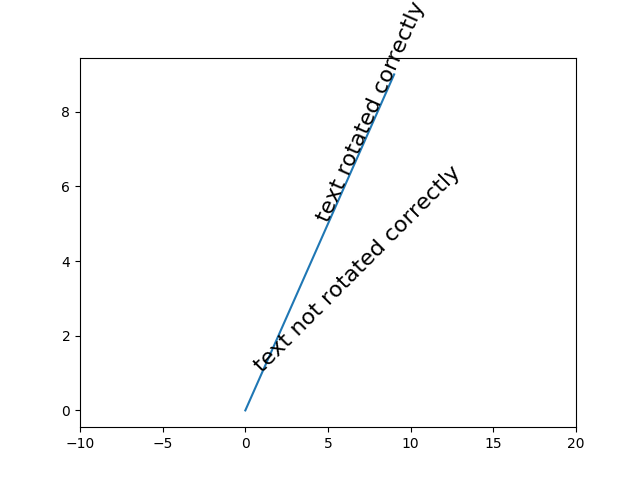# Text Rotation Relative To Line¶

Text objects in matplotlib are normally rotated with respect to the screen coordinate system (i.e., 45 degrees rotation plots text along a line that is in between horizontal and vertical no matter how the axes are changed). However, at times one wants to rotate text with respect to something on the plot. In this case, the correct angle won’t be the angle of that object in the plot coordinate system, but the angle that that object APPEARS in the screen coordinate system. This angle is found by transforming the angle from the plot to the screen coordinate system, as shown in the example below.```import matplotlib.pyplot as plt
import numpy as np

# Plot diagonal line (45 degrees)
h = plt.plot(np.arange(0, 10), np.arange(0, 10))

# set limits so that it no longer looks on screen to be 45 degrees
plt.xlim([-10, 20])

# Locations to plot text
l1 = np.array((1, 1))
l2 = np.array((5, 5))

# Rotate angle
angle = 45
trans_angle = plt.gca().transData.transform_angles(np.array((45,)),
l2.reshape((1, 2)))

# Plot text
th1 = plt.text(l1, l1, 'text not rotated correctly', fontsize=16,
rotation=angle, rotation_mode='anchor')
th2 = plt.text(l2, l2, 'text rotated correctly', fontsize=16,
rotation=trans_angle, rotation_mode='anchor')

plt.show()
```

Gallery generated by Sphinx-Gallery